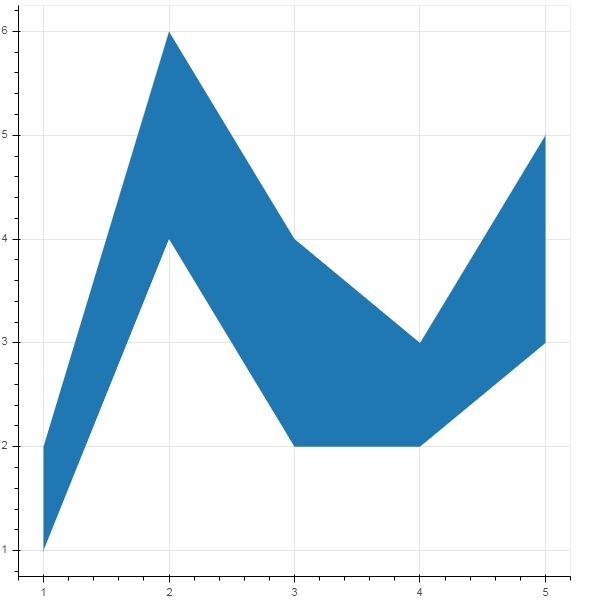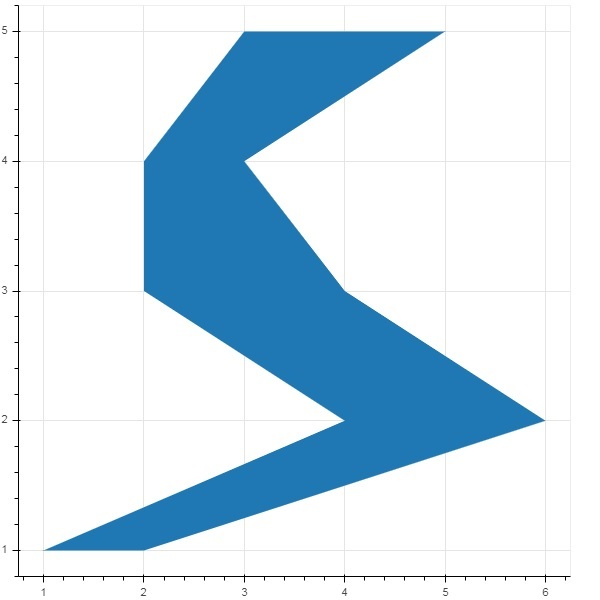# Bokeh - Area Plots

Area plots are filled regions between two series that share a common index. Bokeh's Figure class has two methods as follows −

## varea()

Output of the varea() method is a vertical directed area that has one x coordinate array, and two y coordinate arrays, y1 and y2, which will be filled between.

 1 x The x-coordinates for the points of the area. 2 y1 The y-coordinates for the points of one side of the area. 3 y2 The y-coordinates for the points of the other side of the area.

### Example

```from bokeh.plotting import figure, output_file, show
fig = figure()
x = [1, 2, 3, 4, 5]
y1 = [2, 6, 4, 3, 5]
y2 = [1, 4, 2, 2, 3]
fig.varea(x = x,y1 = y1,y2 = y2)
output_file('area.html')
show(fig)
```

### Output## harea()

The harea() method on the other hand needs x1, x2 and y parameters.

 1 x1 The x-coordinates for the points of one side of the area. 2 x2 The x-coordinates for the points of the other side of the area. 3 y The y-coordinates for the points of the area.

### Example

```from bokeh.plotting import figure, output_file, show
fig = figure()
y = [1, 2, 3, 4, 5]
x1 = [2, 6, 4, 3, 5]
x2 = [1, 4, 2, 2, 3]
fig.harea(x1 = x1,x2 = x2,y = y)
output_file('area.html')
show(fig)
```

### Output